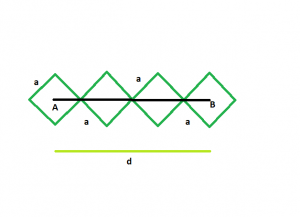# Find the side of the squares which are inclined diagonally and lined in a row

Given here are n squares which are inclined and touch each other externally at vertices, and are lined up in a row.The distance between the centers of the first and last square is given.The squares have equal side length.The task is to find the side of each square.

Examples:

Input :d = 42, n = 4
Output :The side of each square is 9.899

Input :d = 54, n = 7
Output :The side of each square is 6.364

## Recommended: Please try your approach on {IDE} first, before moving on to the solution.Approach:

There are n squares each having side of length a and the distance between the first and last squares is equal to d. From the figure, it is clear that they are connected by diagonals. Length of each diagonal is equal to a√2.
For the first and last square, only half of the diagonal is covered under the length d.For rest of the (n-2) squares, the complete diagonal is covered in d. Hence the relation between a and d is as follows:

a/√2 + a/√2 + (n-2)*a√2 = d
=> a√2 + √2na – 2a√2 = d
=> n√2a – a√2 = d
=> a = d/((n-1)*(√2))
Side of the square = distance between centers/((no. of squares-1) * sqrt(2)).

Below is the implementation of the above approach:

## C++

 `// C++ program to find side of the squares ` `// inclined and touch each other externally ` `// at vertices and are lined in a row ` `// and distance between the ` `// centers of first and last squares is given ` `#include ` `using` `namespace` `std; ` ` `  `void` `radius(``double` `n, ``double` `d) ` `{ ` `    ``cout << ``"The side of each square is "` `         ``<< d / ((n - 1) * ``sqrt``(2)) << endl; ` `} ` ` `  `// Driver code ` `int` `main() ` `{ ` `    ``double` `d = 42, n = 4; ` `    ``radius(n, d); ` `    ``return` `0; ` `} `

## Java

 `// Java program to find side of the squares ` `// inclined and touch each other externally ` `// at vertices and are lined in a row ` `// and distance between the ` `// centers of first and last squares is given ` `import` `java.io.*; ` ` `  `class` `GFG  ` `{ ` ` `  ` `  `static` `void` `radius(``double` `n, ``double` `d) ` `{ ` `    ``System.out.print( ``"The side of each square is "``+ ` `        ``d / ((n - ``1``) * Math.sqrt(``2``))); ` `} ` ` `  `// Driver code ` `public` `static` `void` `main (String[] args)  ` `{ ` `    ``double` `d = ``42``, n = ``4``; ` `    ``radius(n, d); ` `} ` `} ` ` `  `// This code is contributed by anuj_67.. `

## Python3

 `# Python program to find side of the squares ` `# inclined and touch each other externally ` `# at vertices and are lined in a row ` `# and distance between the ` `# centers of first and last squares is given ` ` `  `def` `radius(n, d): ` ` `  `    ``print``(``"The side of each square is "``, ` `        ``d ``/` `((n ``-` `1``) ``*` `(``2``*``*``(``1``/``2``)))); ` ` `  `# Driver code ` `d ``=` `42``; n ``=` `4``; ` `radius(n, d); ` ` `  `# This code is contributed by Rajput-Ji `

## C#

 `// C# program to find side of the squares ` `// inclined and touch each other externally ` `// at vertices and are lined in a row ` `// and distance between the ` `// centers of first and last squares is given ` `using` `System; ` ` `  `class` `GFG  ` `{ ` ` `  ` `  `static` `void` `radius(``double` `n, ``double` `d) ` `{ ` `    ``Console.WriteLine( ``"The side of each square is "``+ ` `        ``d / ((n - 1) * Math.Sqrt(2))); ` `} ` ` `  `// Driver code ` `public` `static` `void` `Main ()  ` `{ ` `    ``double` `d = 42, n = 4; ` `    ``radius(n, d); ` `} ` `} ` ` `  `// This code is contributed by anuj_67.. `

Output:

```The side of each square is 9.89949
```

Time Complexity : O(1)

My Personal Notes arrow_drop_upCheck out this Author's contributed articles.

If you like GeeksforGeeks and would like to contribute, you can also write an article using contribute.geeksforgeeks.org or mail your article to contribute@geeksforgeeks.org. See your article appearing on the GeeksforGeeks main page and help other Geeks.

Please Improve this article if you find anything incorrect by clicking on the "Improve Article" button below.

Improved By : vt_m, Rajput-Ji

Article Tags :
Practice Tags :

Be the First to upvote.

Please write to us at contribute@geeksforgeeks.org to report any issue with the above content.